﻿ Fibonacci数列及其推广形式的正整数表示 Representation of Natural Numbers Using Generalized Fibonacci Sequence

Vol.05 No.02(2016), Article ID:17550,7 pages
10.12677/AAM.2016.52029

Representation of Natural Numbers Using Generalized Fibonacci Sequence

Tingting Guo1, Fugang Chao1, Han Ren1,2*

1Department of Mathematics, East China Normal University, Shanghai

2Shanghai Key Laboratory of Pure Mathematics and Mathematical Practice, ShanghaiReceived: Apr. 24th, 2016; accepted: May 10th, 2016; published: May 13th, 2016ABSTRACT

Previously, there were many studies about the problem of representation of natural numbers. But it’s comparatively rare to study the problem based on Fibonacci sequence and its extension. This thesis mainly discussed the feasibility and diversity of this kind of representation. Utilizing enumerating polynomials and binomial codes, we focused on minimal and maximal representations of natural numbers. In addition to Fibonacci sequence, we also studied the situation of Lucas sequence and offered some hypotheses in the case of n-step Fibonacci sequence.

Keywords:Representation of Natural Numbers, Fibonacci Sequence, Enumerating Polynomial, Binomial CodeFibonacci数列及其推广形式的正整数表示

1华东师范大学数学系，上海

2上海市核心数学与实践重点实验室，上海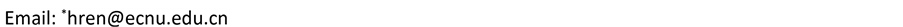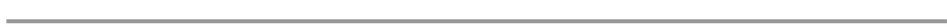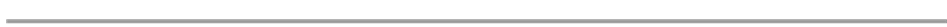1. 引言

Fibonacci数列与许多数学概念联系密切，并具有许多漂亮的性质。人们在许多表面上看似没有任何联系的问题中都发现了它们，使得这一数列成为很基本的一个数学对象。例如：上n阶楼梯，每次只能上1阶或2阶，的不同方法数。就是与Fibonacci数列有相同的初始值和递推关系的数列。一个更有趣的事实是这个正整数的序列，它的通项竟然可以用无理数来表示。

2. 正整数表示的存在性和最多与最少的表示问题3. Fibonacci最少表示的唯一性与其最多表示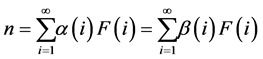Table 1. Enumerating minimal polynomials using Fibonacci numbersTable 2. Recurrence relation of the minimal representation using Fibonacci numbers4. Fibonacci的表示数

5. Lucas的表示Table 3. Enumerating maximal polynomials using Fibonacci numbersTable 4. Recurrence relation of the maximal representation using Fibonacci numbers

6. n代Fibonacci数列的表示Table 5. The number of possibility of representation of n using Fibonacci numbersTable 6. Recurrence relation of the minimal representation using Lucas numbers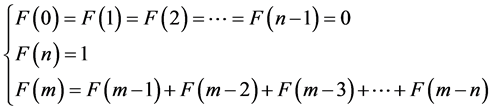Representation of Natural Numbers Using Generalized Fibonacci Sequence[J]. 应用数学进展, 2016, 05(02): 225-231. http://dx.doi.org/10.12677/AAM.2016.52029

1. 1. Hoggatt Jr., V.E. and Chow, B. (1972) Some Theorems on Completeness. Fibonacci Quarterly, 10, 551-554.

2. 2. Ferns, H.H. (1965) On the Representation of Integers as Sums of Distinct Fibonacci Numbers. Fibonacci Quarterly, 3, 21-30.

3. 3. Brown Jr., J.L. (1964) Zeckendorf’s Theorem and Some Applications. Fibonacci Quarterly, 2, 163-168.

4. 4. Bicknell-Johnson, M. and Fielder, D.C. (1999) The Number of Representations of n Using Distinct Fibonacci Numbers, Counted by Recursive Formulas. Fibonacci Quarterly, 37, 47-60.

5. 5. Brown Jr., J.L. (1969) Unique Representations of Integers as Sums of Distinct Lucas Numbers. Fibonacci Quarterly, 7, 243-252.

6. 6. Daykin, D.E. (1969) Representations of Natural Numbers as Sums of Generalized Fibonacci Numbers. Fibonacci Quarterly, 7, 494-510.